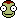• 1

# Designing a GPS constellation - a question for math nerds.

## Question

I'm designing a GPS constellation for KerbalGPS Revived - thanks to USN I understand the geometry of CPA, and that leads me to realize there's a problem:

If the transmitter has a range of 2,500km and I put it in a 2,500km orbit - it's only in range of a given receiver when it's exactly overhead.  The maximum orbit height (yielding horizon-to-horizon coverage) is actually defined by a tangent 2500km long, originating at the receiver.   So my question would be, what is the orbital altitude defined by the endpoint of the tangent?

## Recommended Posts

• 1

Use Pythagoras.

You have a triangle CSR (Kerbin Centre, Satellite and Receiver) which has a right angle at R (because tangent).

So: CS^2 = RS^2 + CR^2. SC (distance of sat to Kerbin centre) is x + 600, SR (distance of sat to receiver) is 2500 and RC (distance of receiver to Kerbin centre) is 600.

(x + 600)^2 = 2500^2 + 600^2. In the end the altitude x of the sat is 1970 km.

Edited by Gaarst
##### Share on other sites

• 0

Well, that turned out to be simpler than I imagined...Thanks!

## Join the conversation

You can post now and register later. If you have an account, sign in now to post with your account.
Note: Your post will require moderator approval before it will be visible.×   Pasted as rich text.   Paste as plain text instead

Only 75 emoji are allowed.